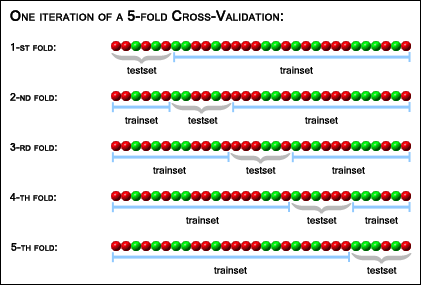# Cross-Validation Explained

There are two types of cross-validation for the classification accuracy estimation:

• K-fold cross-validation
• Leave-one-out cross-validation
They are discussed in the subsections below.

## K-fold Cross-Validation

One iteration of the K-fold cross-validation is performed in the following way: First, a random permutation of the sample set is generated and partitioned into K subsets ("folds") of about equal size. Of the K subsets, a single subset is retained as the validation data for testing the model (this subset is called the "testset"), and the remaining K - 1 subsets together are used as training data ("trainset"). Then a model is trained on the trainset and its accuracy is evaluated on the testset. Model training and evaluation is repeated K times, with each of the K subsets used exactly once as the testset.

The case of a 5-fold cross-validation with 30 samples is illustrated in the picture below:The resulting accuracy estimation depends on the random permutation that was generated in the beginning of the process, because it affects the way the sample set is partitioned. Therefore to obtain a more exact estimation of the accuracy it makes sense to repeat cross-validation several times and take the average of all the accuracy estimations obtained after each iteration as the resulting accuracy estimation.

## Leave-one-out Cross-Validation

As the name suggests, leave-one-out cross-validation involves using a single sample from the original sample set as the validation data, and the remaining samples as the training data. This is repeated such that each sample in the sample set is used exactly once as the validation data. This is the same as K-fold cross-validation where K is equal to the number of samples in the sample set.

There is no need in generating random permutations for leave-one-out cross-validation and in repeating it, because the training and validation datasets for each of the folds are always the same, and therefore the result of the accuracy estimation is determined.

ProClassify User's Guide